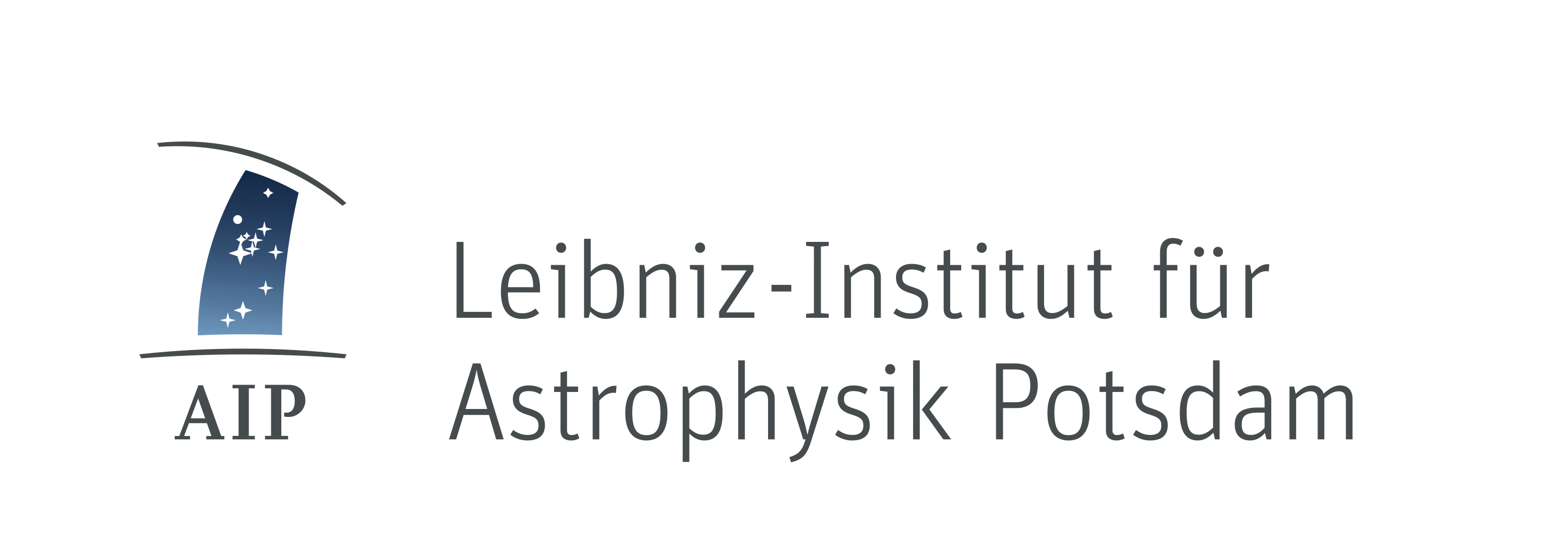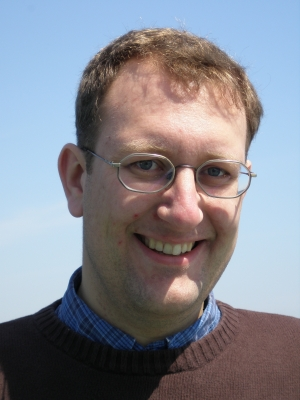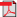Christoph Pfrommer
Back to Lectures overview

## Modern Computational Astrophysics: Concepts and Applications

The objectives of this course are to endow students with the capacity to identify and classify common numerical problems in modern astrophysics. The course aims at an active understanding of numerical methods and algorithms as well as their ranges of applicability. Solving basic astrophysical problems with adequate numerical techniques and determining the range of validity is an essential part of the course. This course (PHY-755) presents theoretical concepts which are then practiced in course PHY-765. A participation of both courses is strongly recommended.

### Lecturers:

Prof. Christoph Pfrommer & Dr. Philipp Girichidis

### Place and Dates:

The lectures take place every Tuesday, at 14:15-15:45 in room 2.28.0.087. The exercise classes take place in the same room immediately after the lectures, 16:00-17:30.

The lectures are based on the revised lecture notes originally written by Prof. Springel (Max-Planck Institute for Astrophysics) and are available as aPDF file (password protected).

### Contents:

• Reviewing basic concepts of numerical simulations (preparatory course)
• Integer arithmetic
• Floating point arithmetic

• Integration of ordinary differential equations
• The Euler method: explicit and implicit
• Runge-Kutta methods and adaptive step sizes
• The leapfrog and symplectic integrators

• Collisionless particle systems
• N-particle ensembles
• Uncorrelated (collisionless) systems
• N-body models of collisionless systems

• Tree algorithms
• Multipole expansion
• Hierarchical grouping
• Tree walk

• The particle-mesh technique
• Mass/charge assignment
• Solving for the potential and force calculation
• Interpolating from the mesh to the particles

• Fourier transform techniques
• Convolution problems
• The discrete Fourier transform
• Non-periodic problems with 'zero padding'

• Basic gas dynamics
• Basic conservation laws
• Euler and Navier-Stokes equations
• Shocks, fluid instabilities and turbulence

• Eulerian hydrodynamics
• Types and solution schemes of partial differential equations
• Advection and the Riemann problem
• Finite volume discretization
• Godunov's method and Riemann solvers
• Extensions to multiple dimensions and high-order accuracy

• Smoothed particle hydrodynamics (SPH)
• Kernel interpolants
• Variational derivation of SPH
• Artificial viscosity

• Diffusion equation
• Explicit method
• Implicit methods

• Paralellization techniques
• Hardware overview
• Shared memory parallelization with OpenMP
• Distributed memory parallelization with MPI

### Homework Assignments

All homework assignments and sample code can be obtained via this link (password protected).

1. Exercise 1 - Ordinary differential equations, due Oct 30, 2018.
2. Exercise 2 - Collisionless particle systems, due Nov 11, 2018.
3. Exercise 3 - Tree algorithms, due Dec 04, 2018.
4. Exercise 4 - Fourier transform and particle mesh techniques, due Dec 11, 2018.
5. Exercise 5 - Sound waves and shocks, due Dec 18, 2018.
6. Exercise 6 - Turbulence and Sedov explosion, due Jan 08, 2019.
7. Exercise 7 - Hyperbolic conservation laws (counts twice), due Jan 29, 2019.
8. Exercise 8 - Diffusion problems, due Feb 5, 2019.

### Credit Points:

Credit points for this lectures can be used for the Master Science Astrophysics Modules PHY-755 and PHY-750.

Grades and credit points are given on the basis of an oral exam. To be eligible for this exam, you need to obtain at least 50% of the points of your homework assignments.